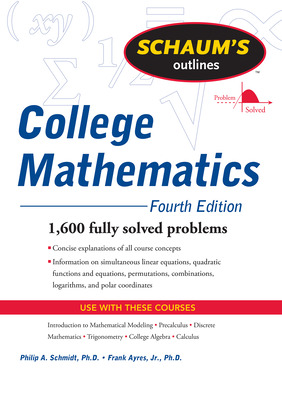# Schaum's Outline of College Mathematics, Fourth Edition

4th Edition
By Philip Schmidt and Frank Ayres
ISBN10: 0071626476
ISBN13: 9780071626477

## Purchase Options

Students, we’re committed to providing you with high-value course solutions backed by great service and a team that cares about your success. See tabs below to explore options and pricing. Don't forget, we accept financial aid and scholarship funds in the form of credit or debit cards.

### Product

Out of stock

The estimated amount of time this product will be on the market is based on a number of factors, including faculty input to instructional design and the prior revision cycle and updates to academic research-which typically results in a revision cycle ranging from every two to four years for this product. Pricing subject to change at any time.

### Program Details

Schaum's Outline of College Mathematics, 4ed
Elements of Algebra
Functions
Graphs of Functions
Linear Equations
Simultaneous Linear Equations
Inequalities
Locus of an Equation
The Straight Line
Families of Straight Lines
The Circle
Arithmetic and Geometric Progressions
Infinite Geometric Series
Mathematical Induction
The Binomial Theorem
Permutations
Combinations
Probability
Determinants of Order Two and Three
Determinants of Order
Systems of Linear Equations
Introduction to Transformational Geometry
Angles and Arc Length
Trigonometric Functions of a General Angle
Trigonometric Functions of an Acute Angle
Reduction to Functions of Positive Acute Angles
Graphs of the Trigonometric Functions
Fundamental Trigonometric Relations and Identities
Trigonometric Functions of Two Angles
Sum, Difference, and Product Trigonometric Formulas
Oblique Triangles
Inverse Trigonometric Functions
Trigonometric Equations
Complex Numbers
The Conic Sections
Transformations of Coordinate
Points in Space
Logarithms
Power, Exponential, and Logarithmic Curves
Polynomial Equations, Rational Roots
Irrational Roots of Polynomial Equations
Graphs of Polynomials
Parametric Equations
The Derivative
Differentiation of Algebraic Expressions
Applications of Derivatives
Integration
Infinite Sequences
Infinite Series
Power Series
Polar Coordinates
Introduction to the Graphing Calculator
The Number System of Algebra
Mathematical Modeling